# Maharashtra (MSBSHSE) SSC Board Question Paper For Class 10 Maths Paper 2 (Algebra) 2016 In PDF

## Maharashtra SSC Maths Algebra (Paper 2) Exam Question Paper 2016 with Solutions – Free Download

MSBSHSE (Maharashtra board) SSC Exam Class 10 Maths 2016 Algebra Question Paper with solutions are given at BYJU’S, with detailed explanations. The solutions of all the previous year question papers of MSBSHSE Class 10 Maths are available in a downloadable pdf format, along with the scrollable images at BYJU’S. All these solutions are prepared by considering all the guidelines of Maharashtra board in terms of necessary steps that have specific marks. It is important for the students to practise all the Geometry and Algebra concepts separately, without leaving any subchapters of these units, since the SSC exam has separate papers for those concepts.

### MSBSHSE Class 10 Mathematics Question Paper 2016 Algebra Paper with Solutions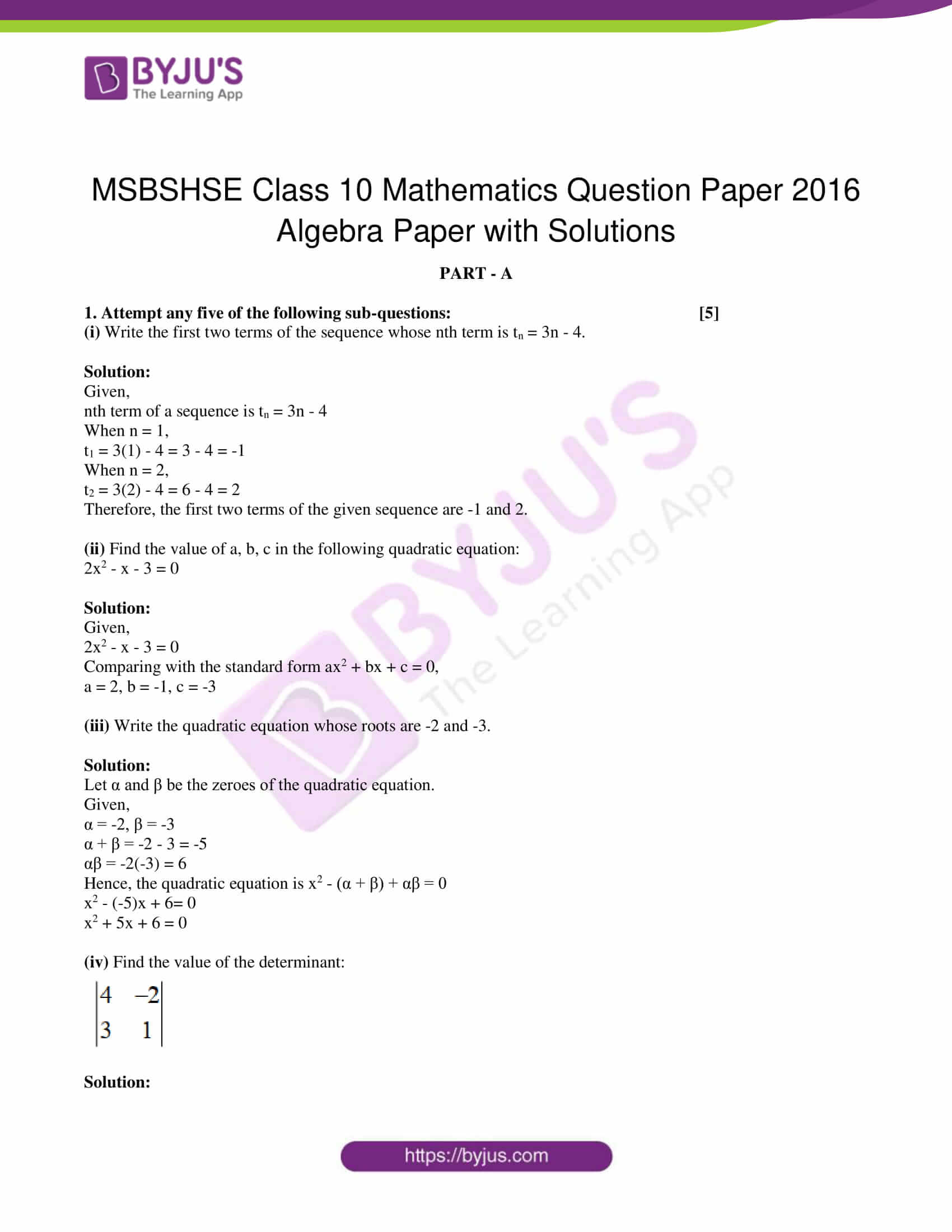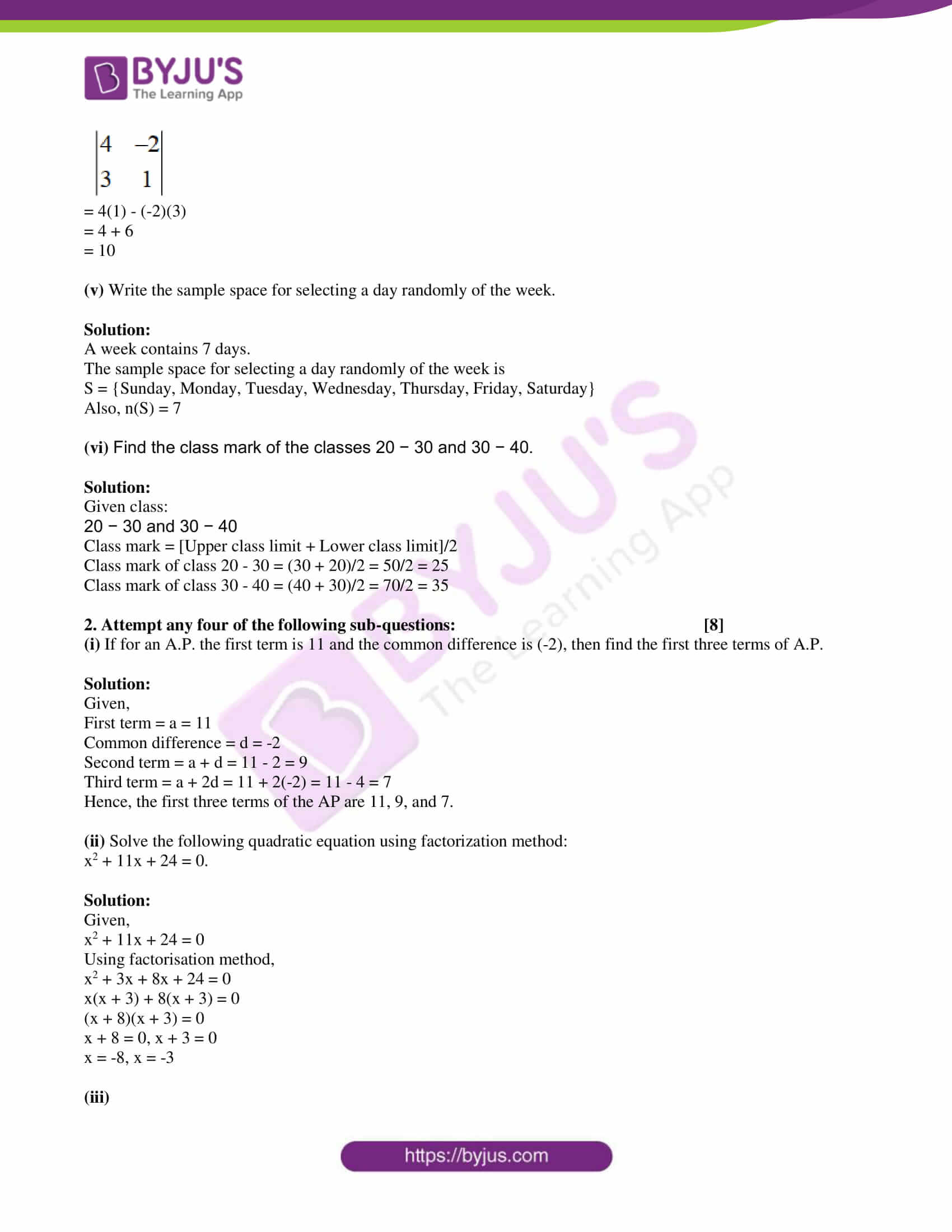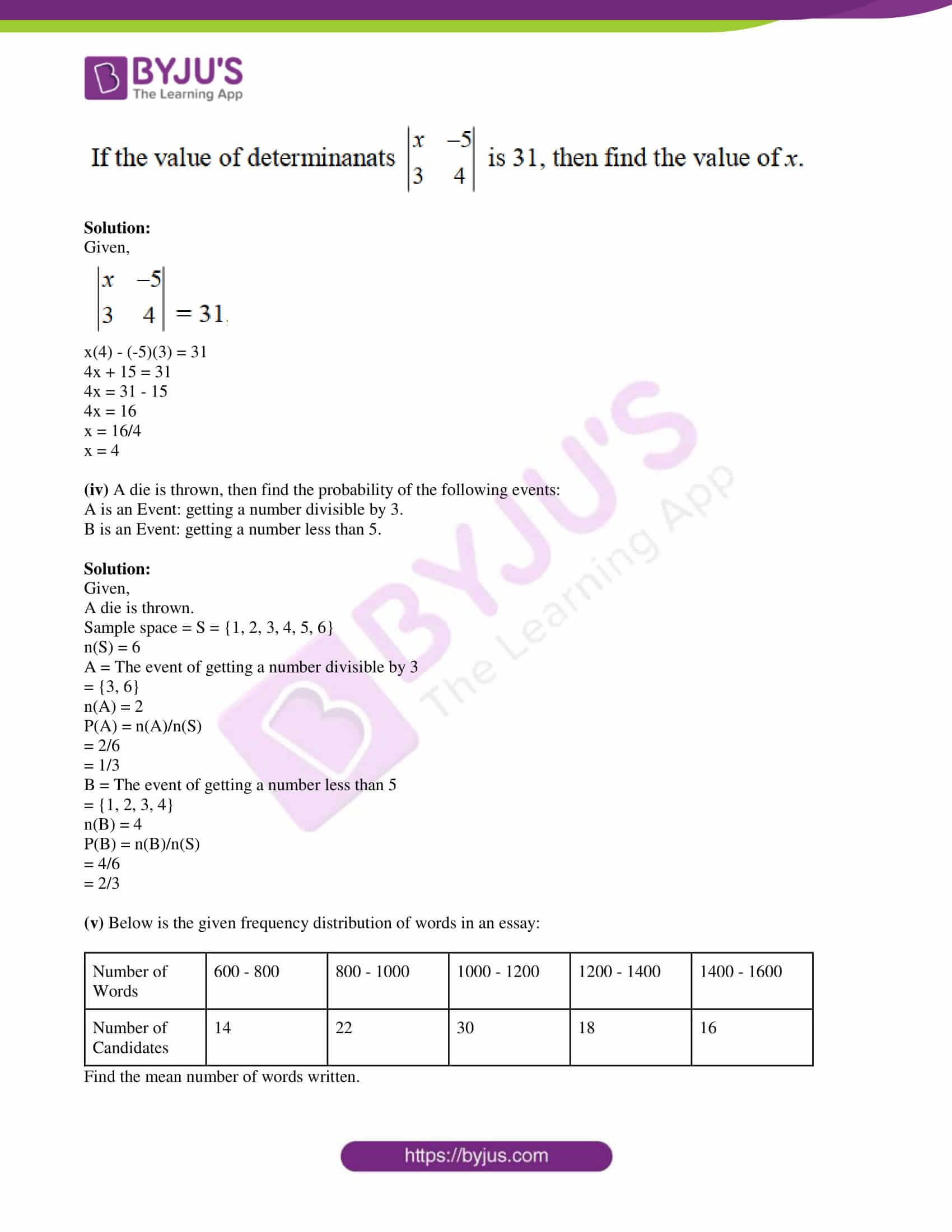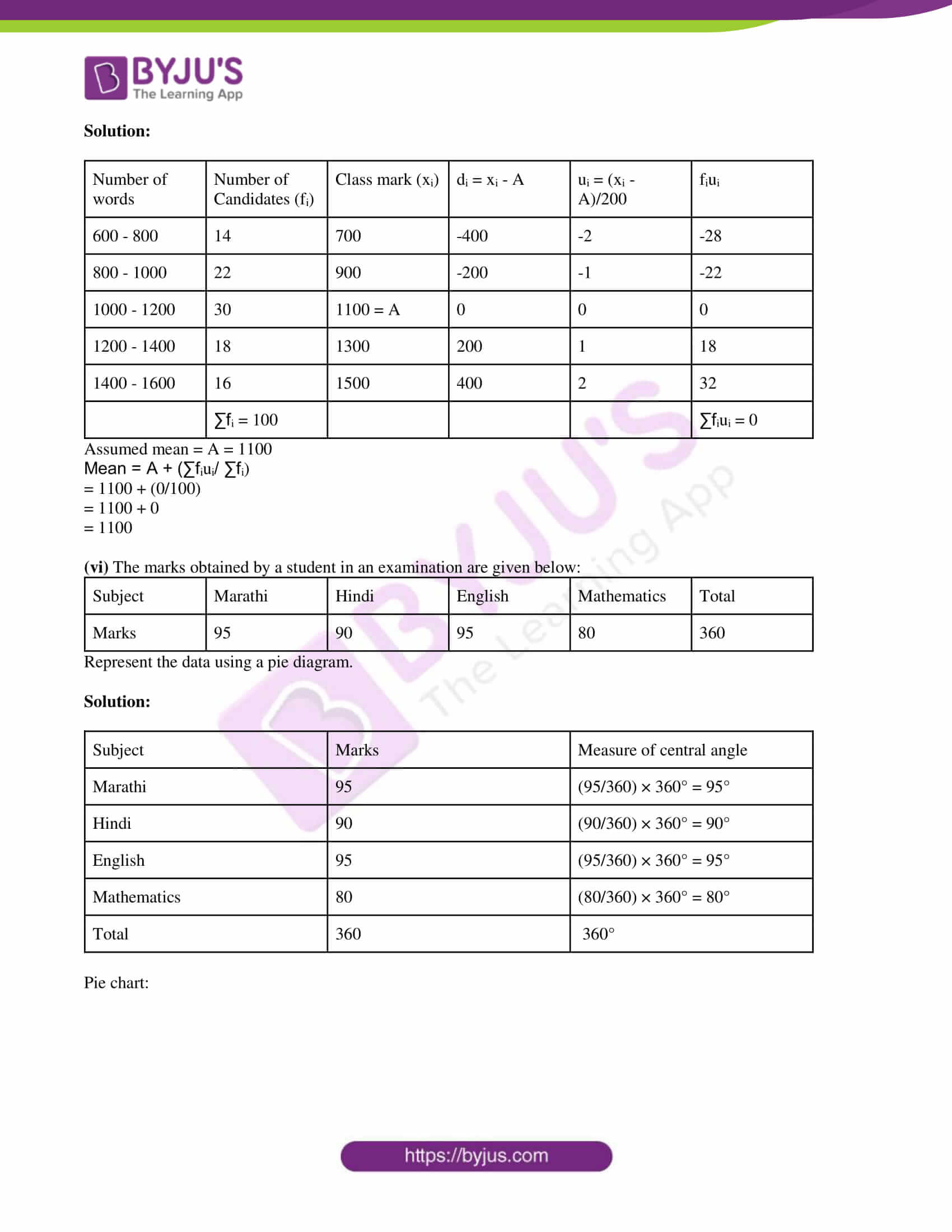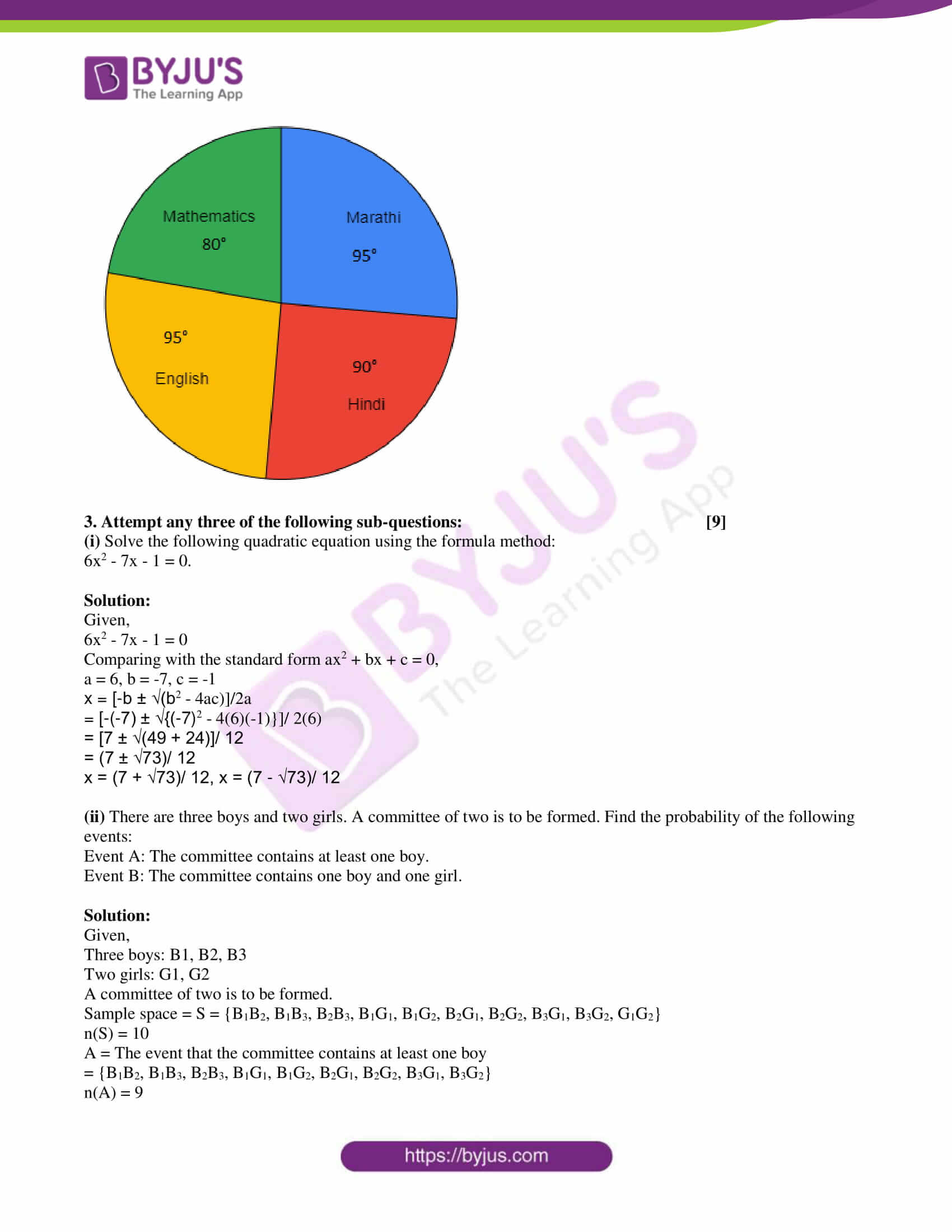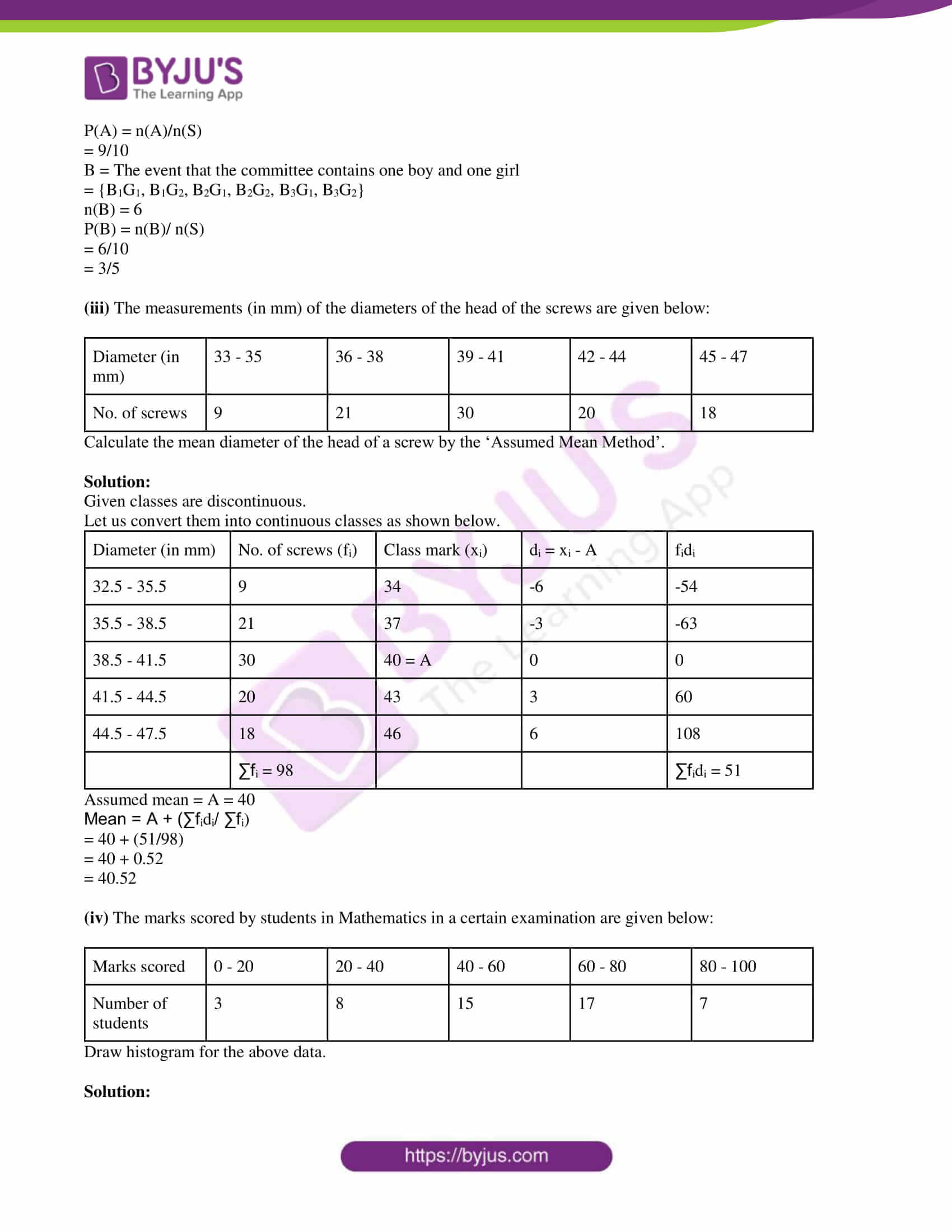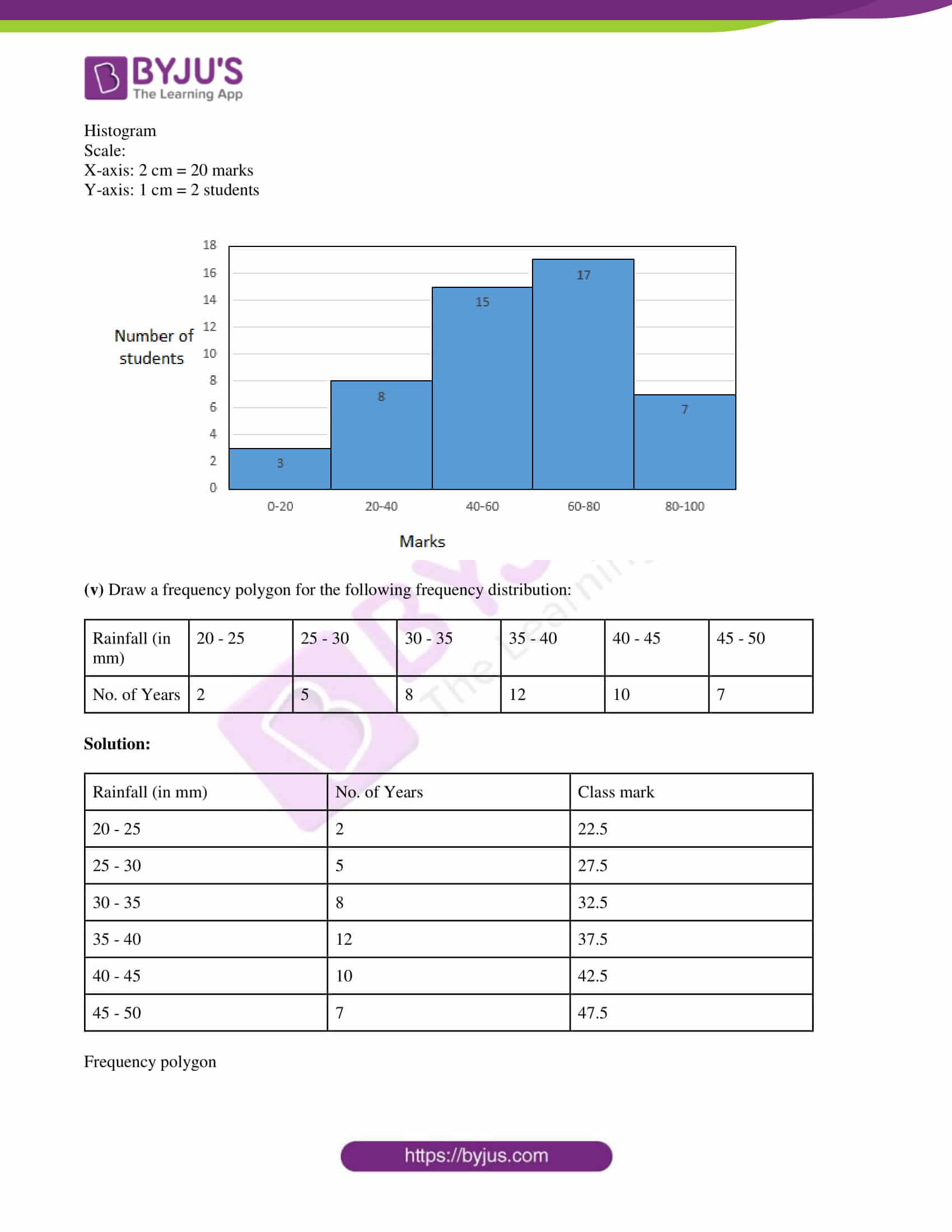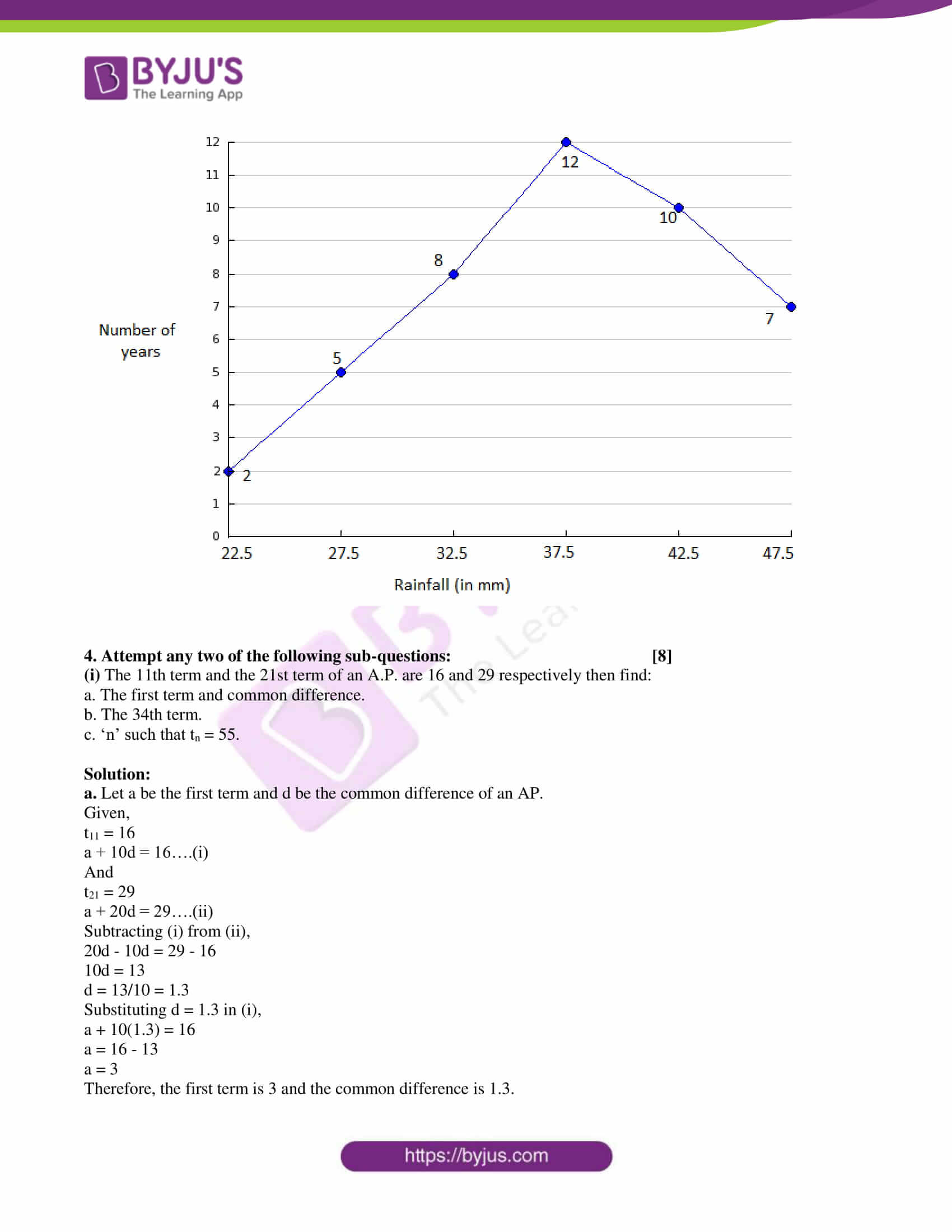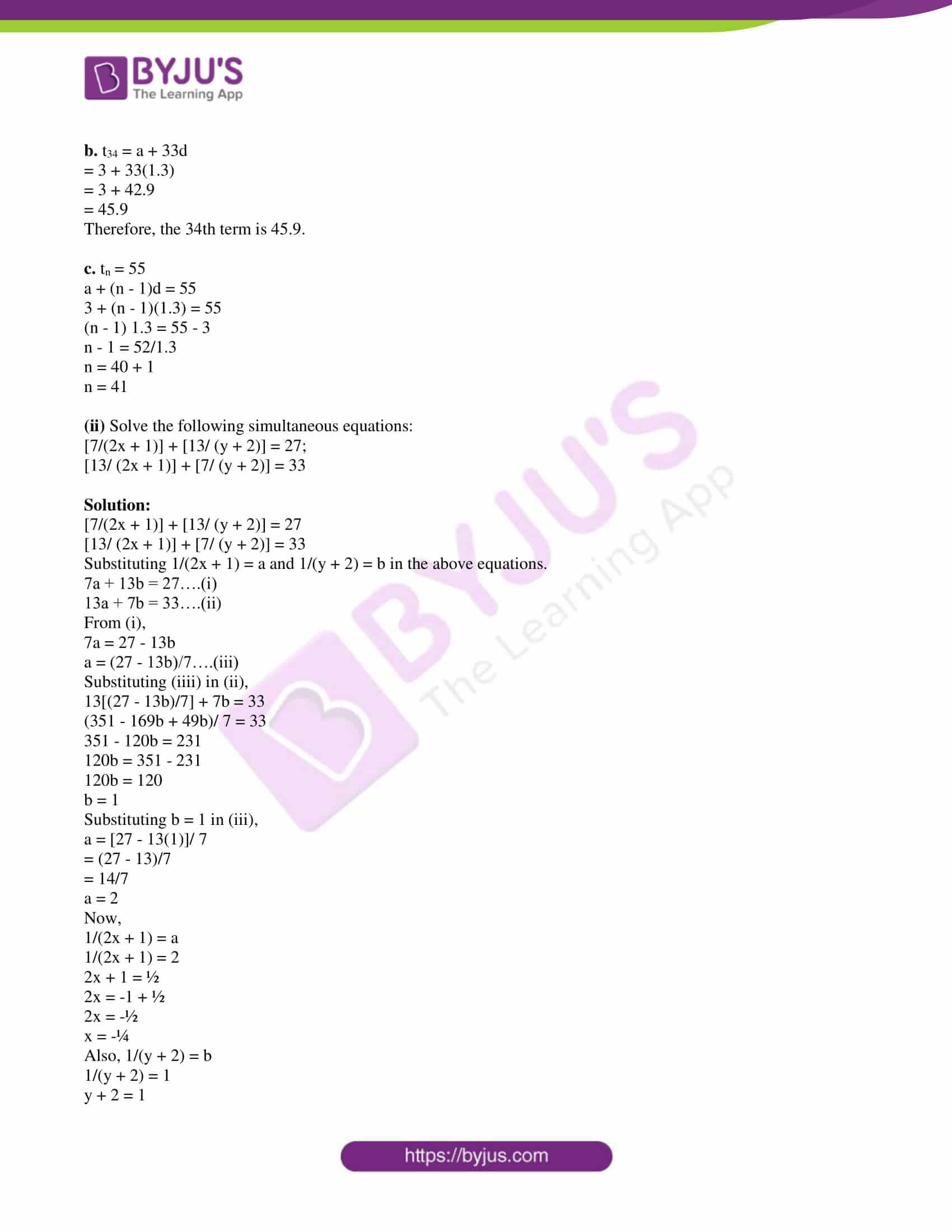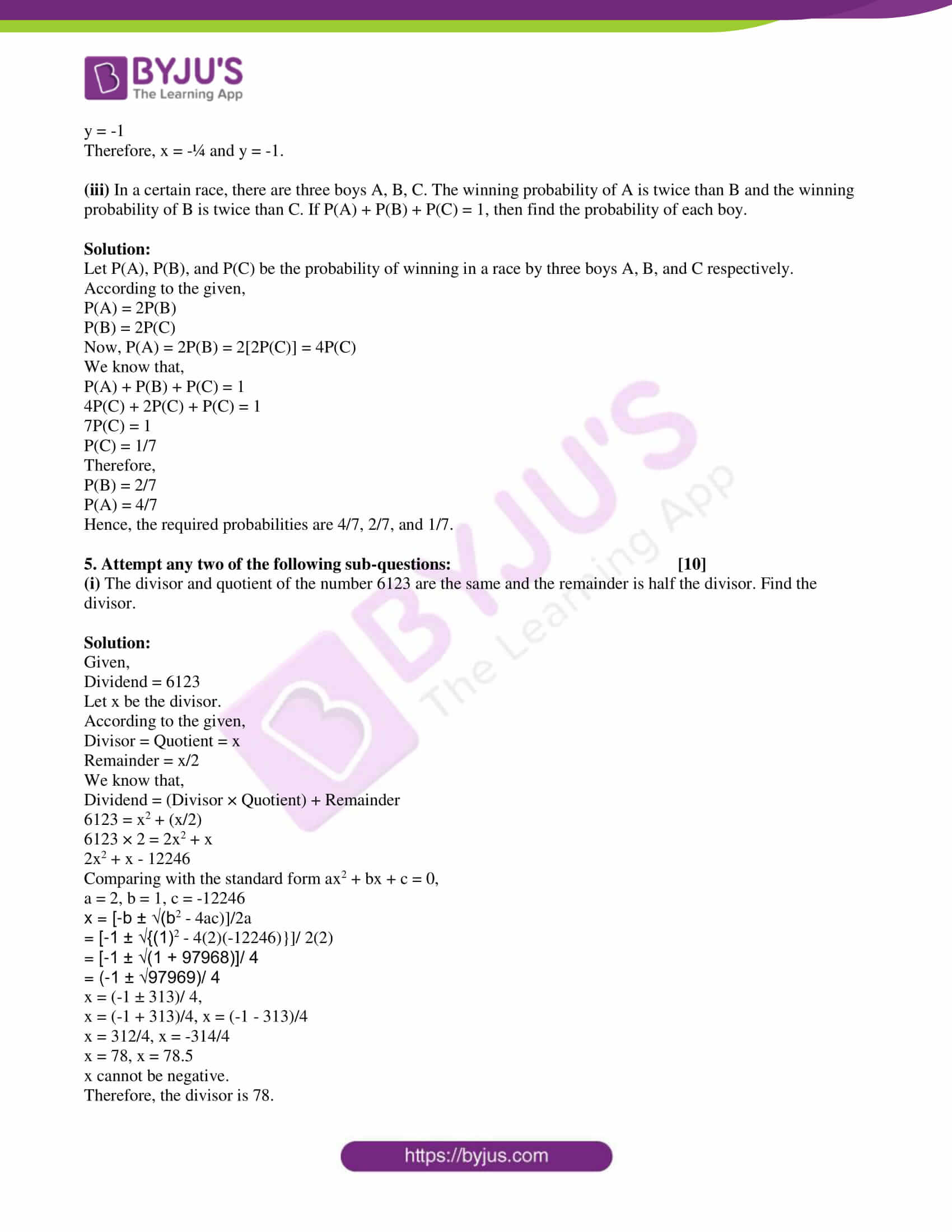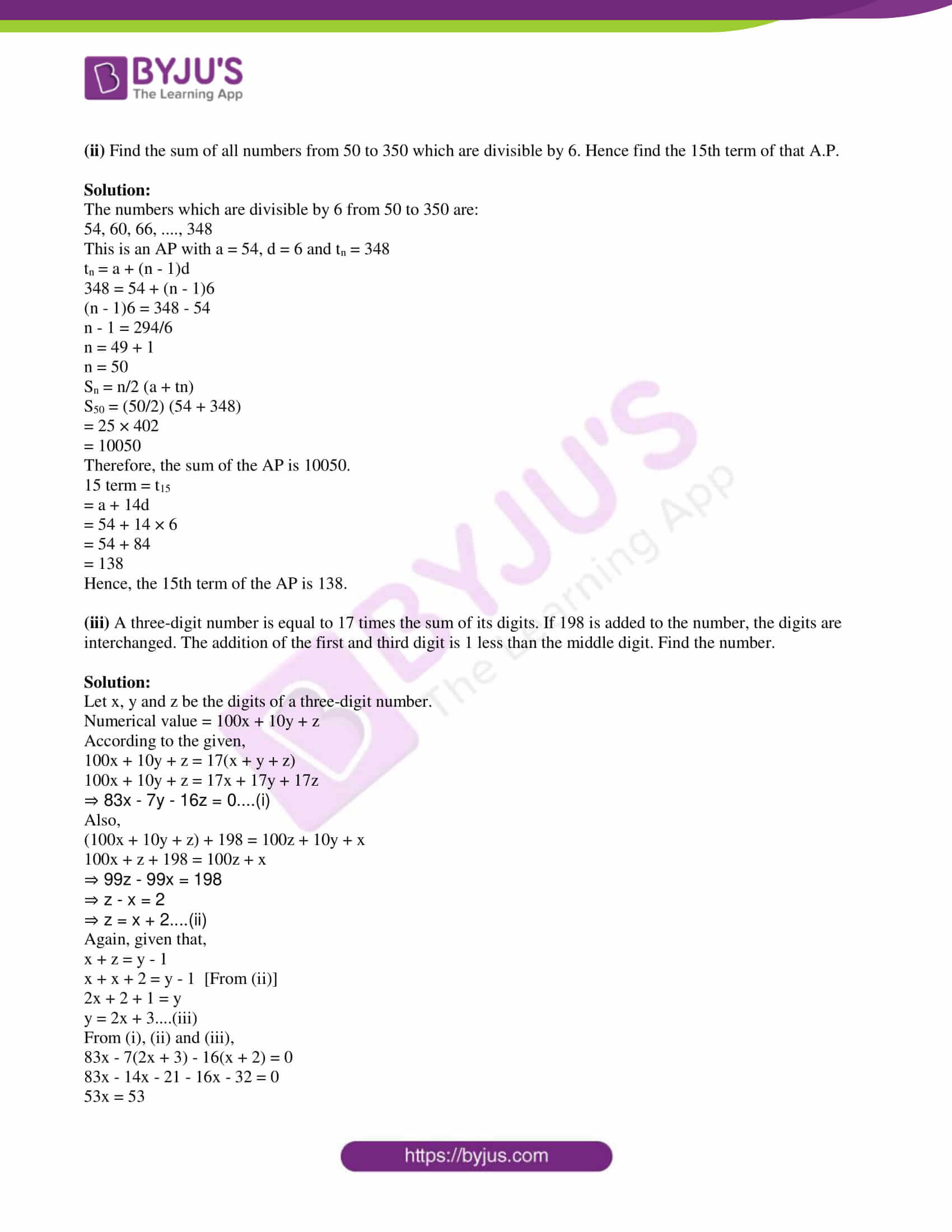PART – A

1. Attempt any five of the following sub-questions: 

(i) Write the first two terms of the sequence whose nth term is tn = 3n – 4.

Solution:

Given,

nth term of a sequence is tn = 3n – 4

When n = 1,

t1 = 3(1) – 4 = 3 – 4 = -1

When n = 2,

t2 = 3(2) – 4 = 6 – 4 = 2

Therefore, the first two terms of the given sequence are -1 and 2.

(ii) Find the value of a, b, c in the following quadratic equation:

2x2 – x – 3 = 0

Solution:

Given,

2x2 – x – 3 = 0

Comparing with the standard form ax2 + bx + c = 0,

a = 2, b = -1, c = -3

(iii) Write the quadratic equation whose roots are -2 and -3.

Solution:

Let α and β be the zeroes of the quadratic equation.

Given,

α = -2, β = -3

α + β = -2 – 3 = -5

αβ = -2(-3) = 6

Hence, the quadratic equation is x2 – (α + β) + αβ = 0

x2 – (-5)x + 6= 0

x2 + 5x + 6 = 0

(iv) Find the value of the determinant: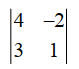Solution:= 4(1) – (-2)(3)

= 4 + 6

= 10

(v) Write the sample space for selecting a day randomly of the week.

Solution:

A week contains 7 days.

The sample space for selecting a day randomly of the week is

S = {Sunday, Monday, Tuesday, Wednesday, Thursday, Friday, Saturday}

Also, n(S) = 7

(vi) Find the class mark of the classes 20 − 30 and 30 − 40.

Solution:

Given class:

20 − 30 and 30 − 40

Class mark = [Upper class limit + Lower class limit]/2

Class mark of class 20 – 30 = (30 + 20)/2 = 50/2 = 25

Class mark of class 30 – 40 = (40 + 30)/2 = 70/2 = 35

2. Attempt any four of the following sub-questions: 

(i) If for an A.P. the first term is 11 and the common difference is (-2), then find the first three terms of A.P.

Solution:

Given,

First term = a = 11

Common difference = d = -2

Second term = a + d = 11 – 2 = 9

Third term = a + 2d = 11 + 2(-2) = 11 – 4 = 7

Hence, the first three terms of the AP are 11, 9, and 7.

(ii) Solve the following quadratic equation using factorization method:

x2 + 11x + 24 = 0.

Solution:

Given,

x2 + 11x + 24 = 0

Using factorisation method,

x2 + 3x + 8x + 24 = 0

x(x + 3) + 8(x + 3) = 0

(x + 8)(x + 3) = 0

x + 8 = 0, x + 3 = 0

x = -8, x = -3

(iii)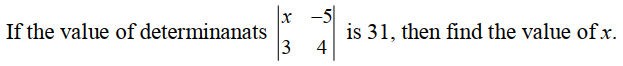Solution:

Given,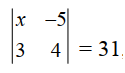x(4) – (-5)(3) = 31

4x + 15 = 31

4x = 31 – 15

4x = 16

x = 16/4

x = 4

(iv) A die is thrown, then find the probability of the following events:

A is an Event: getting a number divisible by 3.

B is an Event: getting a number less than 5.

Solution:

Given,

A die is thrown.

Sample space = S = {1, 2, 3, 4, 5, 6}

n(S) = 6

A = The event of getting a number divisible by 3

= {3, 6}

n(A) = 2

P(A) = n(A)/n(S)

= 2/6

= 1/3

B = The event of getting a number less than 5

= {1, 2, 3, 4}

n(B) = 4

P(B) = n(B)/n(S)

= 4/6

= 2/3

(v) Below is the given frequency distribution of words in an essay:

 Number of Words 600 – 800 800 – 1000 1000 – 1200 1200 – 1400 1400 – 1600 Number of Candidates 14 22 30 18 16

Find the mean number of words written.

Solution:

 Number of words Number of Candidates (fi) Class mark (xi) di = xi – A ui = (xi – A)/200 fiui 600 – 800 14 700 -400 -2 -28 800 – 1000 22 900 -200 -1 -22 1000 – 1200 30 1100 = A 0 0 0 1200 – 1400 18 1300 200 1 18 1400 – 1600 16 1500 400 2 32 ∑fi = 100 ∑fiui = 0

Assumed mean = A = 1100

Mean = A + (∑fiui/ ∑fi)

= 1100 + (0/100)

= 1100 + 0

= 1100

(vi) The marks obtained by a student in an examination are given below:

 Subject Marathi Hindi English Mathematics Total Marks 95 90 95 80 360

Represent the data using a pie diagram.

Solution:

 Subject Marks Measure of central angle Marathi 95 (95/360) × 360° = 95° Hindi 90 (90/360) × 360° = 90° English 95 (95/360) × 360° = 95° Mathematics 80 (80/360) × 360° = 80° Total 360 360°

Pie chart: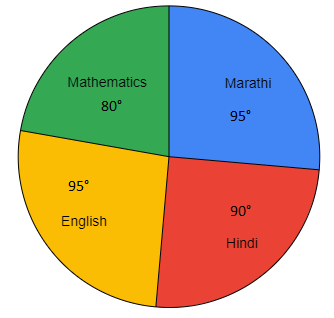3. Attempt any three of the following sub-questions: 

(i) Solve the following quadratic equation using the formula method:

6x2 – 7x – 1 = 0.

Solution:

Given,

6x2 – 7x – 1 = 0

Comparing with the standard form ax2 + bx + c = 0,

a = 6, b = -7, c = -1

x = [-b ± √(b2 – 4ac)]/2a

= [-(-7) ± √{(-7)2 – 4(6)(-1)}]/ 2(6)

= [7 ± √(49 + 24)]/ 12

= (7 ± √73)/ 12

x = (7 + √73)/ 12, x = (7 – √73)/ 12

(ii) There are three boys and two girls. A committee of two is to be formed. Find the probability of the following events:

Event A: The committee contains at least one boy.

Event B: The committee contains one boy and one girl.

Solution:

Given,

Three boys: B1, B2, B3

Two girls: G1, G2

A committee of two is to be formed.

Sample space = S = {B1B2, B1B3, B2B3, B1G1, B1G2, B2G1, B2G2, B3G1, B3G2, G1G2}

n(S) = 10

A = The event that the committee contains at least one boy

= {B1B2, B1B3, B2B3, B1G1, B1G2, B2G1, B2G2, B3G1, B3G2}

n(A) = 9

P(A) = n(A)/n(S)

= 9/10

B = The event that the committee contains one boy and one girl

= {B1G1, B1G2, B2G1, B2G2, B3G1, B3G2}

n(B) = 6

P(B) = n(B)/ n(S)

= 6/10

= 3/5

(iii) The measurements (in mm) of the diameters of the head of the screws are given below:

 Diameter (in mm) 33 – 35 36 – 38 39 – 41 42 – 44 45 – 47 No. of screws 9 21 30 20 18

Calculate the mean diameter of the head of a screw by the ‘Assumed Mean Method’.

Solution:

Given classes are discontinuous.

Let us convert them into continuous classes as shown below.

 Diameter (in mm) No. of screws (fi) Class mark (xi) di = xi – A fidi 32.5 – 35.5 9 34 -6 -54 35.5 – 38.5 21 37 -3 -63 38.5 – 41.5 30 40 = A 0 0 41.5 – 44.5 20 43 3 60 44.5 – 47.5 18 46 6 108 ∑fi = 98 ∑fidi = 51

Assumed mean = A = 40

Mean = A + (∑fidi/ ∑fi)

= 40 + (51/98)

= 40 + 0.52

= 40.52

(iv) The marks scored by students in Mathematics in a certain examination are given below:

 Marks scored 0 – 20 20 – 40 40 – 60 60 – 80 80 – 100 Number of students 3 8 15 17 7

Draw histogram for the above data.

Solution:

Histogram

Scale:

X-axis: 2 cm = 20 marks

Y-axis: 1 cm = 2 students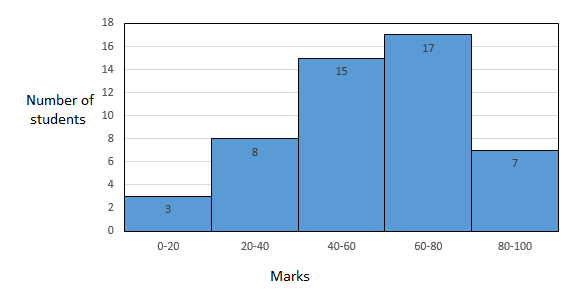(v) Draw a frequency polygon for the following frequency distribution:

 Rainfall (in mm) 20 – 25 25 – 30 30 – 35 35 – 40 40 – 45 45 – 50 No. of Years 2 5 8 12 10 7

Solution:

 Rainfall (in mm) No. of Years Class mark 20 – 25 2 22.5 25 – 30 5 27.5 30 – 35 8 32.5 35 – 40 12 37.5 40 – 45 10 42.5 45 – 50 7 47.5

Frequency polygon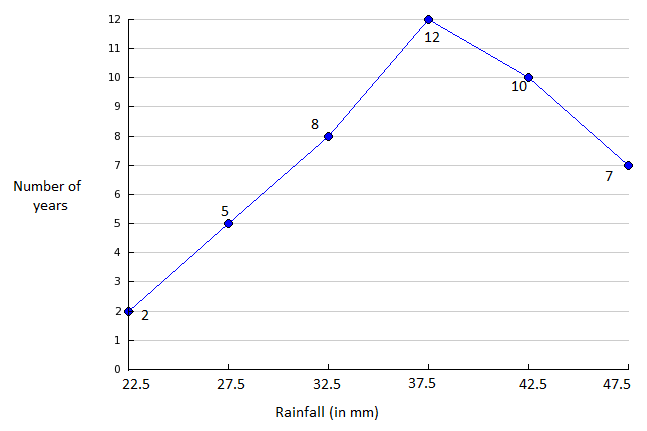4. Attempt any two of the following sub-questions: 

(i) The 11th term and the 21st term of an A.P. are 16 and 29 respectively then find:

a. The first term and common difference.

b. The 34th term.

c. ‘n’ such that tn = 55.

Solution:

a. Let a be the first term and d be the common difference of an AP.

Given,

t11 = 16

a + 10d = 16….(i)

And

t21 = 29

a + 20d = 29….(ii)

Subtracting (i) from (ii),

20d – 10d = 29 – 16

10d = 13

d = 13/10 = 1.3

Substituting d = 1.3 in (i),

a + 10(1.3) = 16

a = 16 – 13

a = 3

Therefore, the first term is 3 and the common difference is 1.3.

b. t34 = a + 33d

= 3 + 33(1.3)

= 3 + 42.9

= 45.9

Therefore, the 34th term is 45.9.

c. tn = 55

a + (n – 1)d = 55

3 + (n – 1)(1.3) = 55

(n – 1) 1.3 = 55 – 3

n – 1 = 52/1.3

n = 40 + 1

n = 41

(ii) Solve the following simultaneous equations:

[7/(2x + 1)] + [13/ (y + 2)] = 27;

[13/ (2x + 1)] + [7/ (y + 2)] = 33

Solution:

[7/(2x + 1)] + [13/ (y + 2)] = 27

[13/ (2x + 1)] + [7/ (y + 2)] = 33

Substituting 1/(2x + 1) = a and 1/(y + 2) = b in the above equations.

7a + 13b = 27….(i)

13a + 7b = 33….(ii)

From (i),

7a = 27 – 13b

a = (27 – 13b)/7….(iii)

Substituting (iiii) in (ii),

13[(27 – 13b)/7] + 7b = 33

(351 – 169b + 49b)/ 7 = 33

351 – 120b = 231

120b = 351 – 231

120b = 120

b = 1

Substituting b = 1 in (iii),

a = [27 – 13(1)]/ 7

= (27 – 13)/7

= 14/7

a = 2

Now,

1/(2x + 1) = a

1/(2x + 1) = 2

2x + 1 = ½

2x = -1 + ½

2x = -½

x = -¼

Also, 1/(y + 2) = b

1/(y + 2) = 1

y + 2 = 1

y = -1

Therefore, x = -¼ and y = -1.

(iii) In a certain race, there are three boys A, B, C. The winning probability of A is twice than B and the winning probability of B is twice than C. If P(A) + P(B) + P(C) = 1, then find the probability of each boy.

Solution:

Let P(A), P(B), and P(C) be the probability of winning in a race by three boys A, B, and C respectively.

According to the given,

P(A) = 2P(B)

P(B) = 2P(C)

Now, P(A) = 2P(B) = 2[2P(C)] = 4P(C)

We know that,

P(A) + P(B) + P(C) = 1

4P(C) + 2P(C) + P(C) = 1

7P(C) = 1

P(C) = 1/7

Therefore,

P(B) = 2/7

P(A) = 4/7

Hence, the required probabilities are 4/7, 2/7, and 1/7.

5. Attempt any two of the following sub-questions: 

(i) The divisor and quotient of the number 6123 are the same and the remainder is half the divisor. Find the divisor.

Solution:

Given,

Dividend = 6123

Let x be the divisor.

According to the given,

Divisor = Quotient = x

Remainder = x/2

We know that,

Dividend = (Divisor × Quotient) + Remainder

6123 = x2 + (x/2)

6123 × 2 = 2x2 + x

2x2 + x – 12246

Comparing with the standard form ax2 + bx + c = 0,

a = 2, b = 1, c = -12246

x = [-b ± √(b2 – 4ac)]/2a

= [-1 ± √{(1)2 – 4(2)(-12246)}]/ 2(2)

= [-1 ± √(1 + 97968)]/ 4

= (-1 ± √97969)/ 4

x = (-1 ± 313)/ 4,

x = (-1 + 313)/4, x = (-1 – 313)/4

x = 312/4, x = -314/4

x = 78, x = 78.5

x cannot be negative.

Therefore, the divisor is 78.

(ii) Find the sum of all numbers from 50 to 350 which are divisible by 6. Hence find the 15th term of that A.P.

Solution:

The numbers which are divisible by 6 from 50 to 350 are:

54, 60, 66, …., 348

This is an AP with a = 54, d = 6 and tn = 348

tn = a + (n – 1)d

348 = 54 + (n – 1)6

(n – 1)6 = 348 – 54

n – 1 = 294/6

n = 49 + 1

n = 50

Sn = n/2 (a + tn)

S50 = (50/2) (54 + 348)

= 25 × 402

= 10050

Therefore, the sum of the AP is 10050.

15 term = t15

= a + 14d

= 54 + 14 × 6

= 54 + 84

= 138

Hence, the 15th term of the AP is 138.

(iii) A three-digit number is equal to 17 times the sum of its digits. If 198 is added to the number, the digits are interchanged. The addition of the first and third digit is 1 less than the middle digit. Find the number.

Solution:

Let x, y and z be the digits of a three digit number.

Numerical value = 100x + 10y + z

According to the given,

100x + 10y + z = 17(x + y + z)

100x + 10y + z = 17x + 17y + 17z

⇒ 83x – 7y – 16z = 0….(i)

Also,

(100x + 10y + z) + 198 = 100z + 10y + x

100x + z + 198 = 100z + x

⇒ 99z – 99x = 198

⇒ z – x = 2

⇒ z = x + 2….(ii)

Again, given that,

x + z = y – 1

x + x + 2 = y – 1 [From (ii)]

2x + 2 + 1 = y

y = 2x + 3….(iii)

From (i), (ii) and (iii),

83x – 7(2x + 3) – 16(x + 2) = 0

83x – 14x – 21 – 16x – 32 = 0

53x = 53

x = 1

Substituting x = 1 in (ii) and (iii),

y = 2(1) + 3 = 2 + 3 = 5

z = 1 + 2 = 3

Hence, the three digit number is 153.